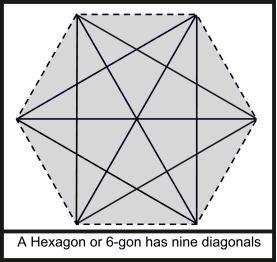favorite We need a little bit of your help to keep things running, click on this banner to learn more
Competitions

# Diagonal

The number of diagonals of an n-gon is not less than N. What is the minimum possible value of n?Input

The input file contains less than 1001 lines of inputs. Each line contains a positive integer N (N1015) that indicates the minimum possible number of diagonals. Input is terminated by a line containing a zero. This line should not be processed.

Output

For each line of input produce one line of output, which contains the output serial number, and also the minimum possible value for n (Number of sides).

Time limit 1 second
Memory limit 128 MiB
Input example #1
```10
100
1000
0
```
Output example #1
```Case 1: 7
Case 2: 16
Case 3: 47
```